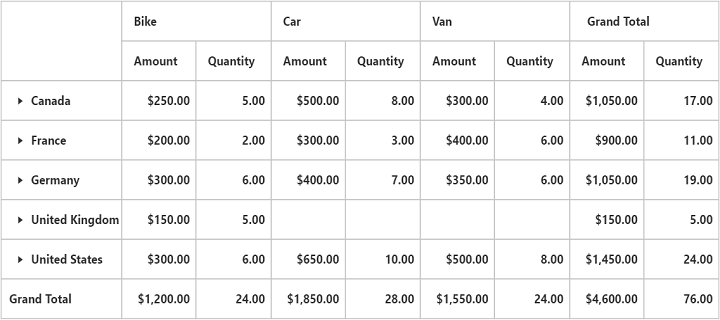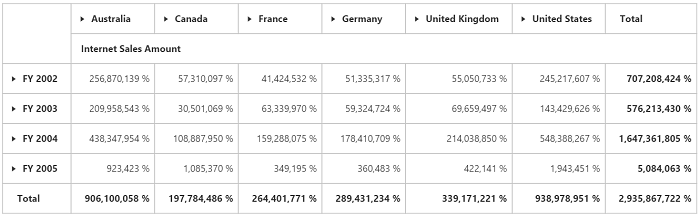# Number Format in EJ 1 Angular PivotGrid

28 Sep 2017 / 2 minutes to read

Allows us to specify the required number format that PivotGrid should use in its values by setting the `format` option. Following number formats that are supported:

• number
• decimal
• currency
• percentage
• date
• time
• scientific
• accounting
• fraction

## RELATIONAL

``````<ej-pivotgrid [dataSource.values]="values">
</ej-pivotgrid>``````
``````//..
export class PivotGridComponent {
//..
constructor() {
//..
this.values= [{ fieldName: "Amount", fieldCaption: "Amount", format: "currency" },
{ fieldName: "Quantity", fieldCaption: "Quantity", format: "decimal" }
],
this.filters= []
}
}``````## OLAP

• ts
• ``````//..
export class PivotGridComponent {
//..
constructor() {
//..
this.values= [{ measures: [{ fieldName: "[Measures].[Internet Sales Amount]",
format: "percent" //Specify the format here
}],
axis: "columns"
}
],
this.filters= []
}
}``````